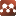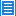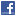## Number Theory## Proof of Riemann Hypothesis and Other Prime Conjectures

Authors: Surajit Ghosh

Riemann hypothesis stands proved in three diﬀerent ways.To prove Riemann hypothesis from the functional equation concept of Delta function is introduced similar to Gamma and Pi function. Zeta values are renormalised to remove the poles of zeta function. Extending sum to product rule fundamental formula of numbers are deﬁned which then helps proving other prime conjectures namely goldbach conjecture, twin prime conjecture etc.